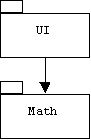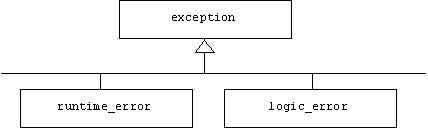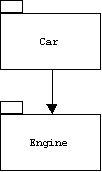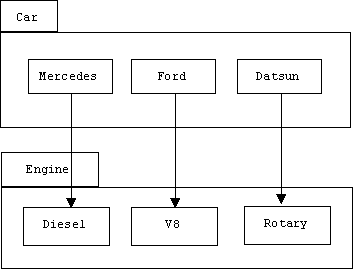# Assume an application is assembled from independently developed modules. How should errors detected by module M be handled? M has four choices:

1. Ignore the error.
2. Flag the error.
3. Display an error message, then gracefully terminate the program.
4. Repair the error, then continue.
The first option is just lazy programming. Ignoring an error will probably cause the program to enter an unstable state and eventually crash. When a program crashes, the operating system may print a cryptic message such as "core dump" or "segmentation violation," or the operating system may also enter an unstable state and eventually crash, requiring a reboot of the computer.
The second option is the one employed by the standard stream library. For example, when we attempt to extract a number from an input file stream, s, that doesn't contain a number, or when we attempt to associate s with a file that doesn't exist, then s enters a fail state, which we can detect by calling s.fail() or by comparing s to the null pointer. If we forget to occasionally check the state of s and repair it if it has failed:
if (s.fail())
{
s.sync();
s.setstate(ios::goodbit);
}
then subsequent operations on s quietly turn into no-ops (i.e., operations that do nothing when they are executed). The program will speed passed subsequent read operations without pausing to extract data. Setting error flags places too much trust and too much burden on client modules.
The third option is ideal when we are debugging an application. When a problem occurs, the programmer wants to know what and where, which is what a good error message should tell him. Graceful termination means the program stops immediately and returns control to the operating system or debugger. We can achieve both of these goals by defining and calling a universal error() function:
inline void error(const string& gripe = "unknown")
{
cerr << "Error, " << gripe << "!\n";
exit(1);
}
The exit() function terminates the program and returns a termination code to the operating system or debugger. Termination code 0 traditionally indicates normal termination, while termination code 1 indicates abnormal termination.
After an application has been released to clients, option 3 is no longer acceptable. Clients will be annoyed if the program terminates unexpectedly and they lose all of their unsaved data. We must use option 4 in this situation. When an error is detected, the program must trace back to the point where the error was introduced. The state of the program at this point must be restored. If the error was caused by a bad user input, for example, then the program might prompt the user to reenter the data.
The problem with option 4 is that just because module M may detect an error, doesn't mean module M can repair the error. In fact, handling the error should be left up to client modules. For example, assume M is a package of mathematical functions called Math and UI is a user interface package that uses Math:Suppose the UI package prompts the user for a number x, then some time later passes x to a log() function defined in Math and displays the result:
double x;
cout << "enter a number: ";
cin >> x;
// etc.
double result = Math::log(x);
cout << "result = " << result << endl;
// etc.
Suppose the user entered a negative number. Of course Math::log() will probably detect that its input is negative and therefore does not have a logarithm. But what should Math::log() do about the bad input? It's the responsibility of the UI package to interact with the user. If the log() function attempts to bypass the UI by reporting the error directly to the user and asking for another input, then it limits its reusability. For example, we wouldn't want to use such a function in a system with a graphical user interface. Also, result may be needed by subsequent calculations just as x may have been needed by previous calculations. Computing the log of a different number may not be relevant in this context.
Perhaps functions in the Math package could return some sort of error tokens to client packages when they detect bad inputs. This idea isn't bad, but it has several drawbacks. First, what should the error token be? For example, if a function returns a double, then we must either agree on a designated double to be used as the error token, which means this number can never be a normal return value. For example, many platforms provide a floating point representation of NaN (Not a Number), which can be referenced in C++ by the expression:
numeric_limits<double>::quiet_NaN
Of course we will have to do this for all possible return types. Alternatively, we could assign output values to a reference parameter and return a Boolean flag indicating if the operation was successful:
bool log(double value, double& result)
{

if (value <= 0) return false; // log failed
result = ...; // calculate log of value
return true; // log succeeded
}
A bigger problem is that clients must check for the error token each time a function is called. If the return value is an error token, then the client must either handle the error or return an error token to its caller. For example, the following function uses our log() function to calculate the maximum data rate of a communication channel with bandwidth bw and signal-to-noise ration snr:
bool dataRate(double snr, double bw, double& result)
{
double factor;
if (!log(1 + snr, factor)) // factor = log of 1 + snr
return false; // dataRate failed
result = bw * factor;
return true; // dataRate succeeded
}

## Catch and Throw

C++, Java, and other languages provide an error reporting mechanism that is similar to the idea of returning error tokens. When a function detects an error, it creates an error token called an exception, then uses the throw operator to "return" the exception to its caller:
double log(double x)
{
if (x <= 0)
{
throw exp;
}
// calculate & return logarithm of x
}
A throw statement is similar to a return statement: it causes log() to terminate immediately. However, the exception returned by the throw statement is an arbitrary object that doesn't need to match the return type of the function. In our example the exception is an instance of an error token class we invented for representing and encapsulating all bad inputs:
{
public:
BadInputException(double x = 0) { irritant = x; }
double getIrritant() { return irritant; }
private:
double irritant;
};
Any other type of object could be thrown instead:
double log(double x)
{
if (x <= 0)
{
throw exp;
}
// calculate & return logarithm of x
}
C++ even allows us to specify the types of exceptions a function might throw. For example, assume we define two exception classes for distinguishing between negative and zero inputs:
class NegInputException: public BadInputexception { ... };
class ZeroInputException: public BadInputexception { ... };
Here is how we can specify that our log() function might throw either exception:
double log(double x) throw (NegInputException, ZeroInputException)
{
if (x < 0) throw NegInputException(x);
if (x == 0) throw ZeroInputException();
// calculate & return logarithm of x
}
Unlike Java, the compiler doesn't require us to declare which exceptions a function might throw. Also, the C++ compiler doesn't generate an error if a function throws a different type of exception than the ones specified. If this happens a system function named unexpected() is automatically called. The default implementation of unexpected() terminates the program.

How does the calling function know if the called function throws an exception, and if so, what should it do? For example, let's re-implement the dataRate() function described earlier. Recall that this function uses our log() function to calculate the maximum data rate of a communication channel with bandwidth bw and signal-to-noise ration snr:
double dataRate(double snr, double bw)
{
double factor = log(1 + snr);
return bw * factor;
}
If snr <= -1, then log(1 + snr) will throw an exception. If this happens, then dataRate() implicitly throws the exception to its caller at the point where log() is called. In particular, dataRate() terminates immediately. The assignment and return statements are never executed.
C++ allows us to specify implicitly thrown exceptions, too:
double dataRate(double snr, double bw)
throw (NegInputException, ZeroInputException)
{
double factor = log(1 + snr);
return bw * factor;
}
In this way an exception explicitly thrown by log() is automatically propagated through the chain of calling functions. But how is the exception ultimately handled? If no function handles the exception, then the system-defined function named terminate() is automatically called. The default implementation of terminate() terminates the program.
If we think we can completely or partially handle some of the exceptions that might be thrown by certain functions, then we call these functions inside of a try block:
try
{
fun1(...);
fun2(...);
// etc.
}
One or more catch blocks immediately follow a try block:
catch(NegInputException e)
{
// handle negative input exception here
}
catch(ZeroInputException e)
{
// handle zero input exception here
}
// etc.
The thrown exception is passed to the catch block through the parameter specified in the parameter list following the reserved word "catch". The parameter type is used to control which handler is invoked when an exception is thrown. For example, if fun1() throws a ZeroInputException, then control is automatically transferred to the first line inside the second catch block.
Suppose our UI package calls dataRate(). Here is how we might handle the negative input exception it throws:
void controlLoop()
{
while(true)
try
{
double bw, snr, rate;
cout << "enter bandwidth: ";
cin >> bw;
cout << "enter signal to noise ratio: ";
cin >> snr;
rate = dataRate(snr, bw);
cout << "maximum data rate = " << rate << " bits/sec\n";
}
catch(NegInputException e)
{
cerr << "signal to noise ratio must not be < -1\n";
cerr << "you entered " << e.getIrritant() - 1 << endl;
}
}
If we enter a signal to noise ratio of –2, then the following //output is produced:
/*
enter bandwidth: 30000
enter signal to noise ratio: -2
signal to noise ratio must not be < -1
you entered -2
enter bandwidth:
*/
If we enter a signal to noise ratio of –1, then dataRate() implicitly throws a ZeroInputException. Because controlLoop() doesn't catch this type of exception, it too implicitly throws the exception.
If controlLoop() wanted to handle both exceptions, we could add an extra catch block for the ZeroInputException:
catch(ZeroInputException e)
{
cerr << "signal to noise ratio must not be -1\n";
}
Alternatively, since ZeroInputException and NegInputException are both derived from BadInputException, we could handle both in a single catch block:
void controlLoop()
{
while(true)
try
{
double bw, snr, rate;
cout << "enter bandwidth: ";
cin >> bw;
cout << "enter signal to noise ratio: ";
cin >> snr;
rate = dataRate(snr, bw);
cout << "maximum data rate = " << rate << " bits/sec\n";
}
{
cerr << "signal to noise ratio must not be <= -1\n";
cerr << "you entered " << e.getIrritant() - 1 << endl;
}
}

## Standard Exceptions

Unlike the flag-setting stream library, the standard template library throws exceptions when things go wrong. The pre-defined exception classes are defined in the <stdexcept> header file:
#include <stdexcept>
The base class for all STL exceptions is exception class.
class exception
{
public:
exception() throw();
exception(const exception& rhs) throw();
exception& operator=(const exception& rhs) throw();
virtual ~exception() throw();
virtual const char *what() const throw();
};
The what() member function normally returns an error message encapsulated by the exception.
The two principle derived classes are logic_error and runtime_error, although there are a number of other exception classes derived from exception:The distinction between these two classes is a little shaky. Presumably, a logic error occurs when a bad thing happens to a bad program. In other words, a logic error is an error in the program, such as passing an invalid argument, using an index that's out of range, attempting to access elements in an empty container, etc. A runtime error occurs when a bad thing happens to a good program. In other words, a runtime error is an error caused by the program's environment, not the program itself, such as an overflow or underflow error. More commonly, runtime errors are thrown when information specified by the user, such as the name of a file to be opened or the index of an array to be accessed, is invalid.
For example, here is how we might deal with the problem of attempting to open a missing or protected file:
ifstream& openFile(const string& fname) throw(runtime_error)
{
ifstream ifs(fname.str());
if (!ifs) throw runtime_error(string("can't open ") + fname);
return ifs;
}
Here is a list of the current exception classes defined in the standard C++ library. Indentation indicated derivation depth:
exception
logic_error
length_error
domain_error
out_of_range
invalid_argument
ios::base::failure
runtime_error
range_error
overflow_error
underflow_error

### The error() function

We can improve the error() function mentioned earlier by controlling its behavior with a global flag indicating if we are in debug or release mode:
// set to false before release build
#define DEBUG_MODE true
In debug mode, the error() function prints an error message and terminates the program. In release mode a runtime error is thrown:
inline void error(const string& gripe = "unknown")
throw (runtime_error)
{
if (DEBUG_MODE)
{
cerr << "Error, " << gripe << "!\n";
exit(1);
}
else // release mode
throw runtime_error(gripe);
}
One problem with this approach is that it prevents us from being more specific about the type of exception we are throwing, hence we can't steer our exception to a particular catch block.

## Example

Assume an application will consist of a provider module called Engine and a client module called Car:Of course the implementer of the Engine module may have no knowledge of the Car client module. Indeed, there may be other client modules.
When problems occur in the Engine module, exceptions are thrown. It's up to the client module to decide how to handle these exceptions. The Engine module can help its clients by providing a carefully designed hierarchy of exceptions. This allows the client to decide the granularity of exception handling. Fore example, should an exception indicating that the oil is low be handled by the same function that handles exceptions indicating that the gas is low? Remember, making this decision is the client's privilege.

### Engines

The Engine subsystem contains several types of engines together with a hierarchy of exceptions representing typical engine problems:We can implement the Engine subsystem as a C++ namespace:
namespace Engine
{
class EngineErr { ... };
class LowOil: public EngineErr { ... };
class LowGas: public EngineErr { ... };
class TooFast: public EngineErr { ... };
class StateErr: public EngineErr { ... };
class Stopped: public StateErr { ... };
class Running: public StateErr { ... };

class V8 { ... };
class Diesel { ... };
class Rotary { ... };
// etc;

} // Engine
Instances of the base class of all engine errors encapsulate error messages:
class EngineErr
{
public:
EngineErr(string s = "unknown error")
{
gripe = string("Warning: ") + s;
}
string what() { return gripe; }
private:
string gripe;
};
We could have derived EngineErr from one of the predefined exception classes such as exception or runtime_error:
class EngineErr: public runtime_error { ... };
This would give clients the option of treating all exceptions the same way.
The LowOilLowGas, and TooFast exceptions encapsulate the current oil, gas, or engine speed, respectively. For example:
class LowOil: public EngineErr
{
public:
LowOil(double amt): EngineErr("oil is low!") { oil = amt; }
double getOil() { return oil; }
private:
double oil;
};
State errors occur when we try to drive a car isn't running or change the oil in a car that is running:
class StateErr: public EngineErr
{
public:
StateErr(string gripe = "Invalid state"): EngineErr(gripe) {}
};
For example, here is the exception thrown when we attempt to change oil in a running car:
class Running:  public StateErr
{
public:
Running(): StateErr("engine running!") {}
};
A typical engine is the V8:
class V8
{
public:

V8(double g = 10, double o = 2);
void print(ostream& os = cout);
void start() throw(LowGas, LowOil, Running);
double getGas() throw(Running);
double getOil() throw(Running);
void run() throw(LowGas, LowOil, Stopped, TooFast);
void stop() throw(Stopped, exception);
private:
double gas; // = gallons of gas
double oil; // = quarts of oil
double rpm; // = engine speed in roations per minute
bool running;
};
The implementation of run() first passes through a gauntlet of throw statements:
void Engine::V8::run() throw(LowGas, LowOil, Stopped, TooFast)
{
if (!running) throw Stopped();
if (gas < .25) throw LowGas(gas);
if (oil < .25) throw LowOil(oil);
if (8000 < rpm) throw TooFast(rpm);
gas *= .5;
oil *= .8;
rpm *= 2;
}
Here is the implementation of getGas():
double Engine::V8::getGas() throw(Running)
{
if (running) throw Running();
gas = 10; // fill it up
return gas;
}

### Cars

The Car subsystem depends on the Engine subsystem:We can represent the Car subsystem as a C++ namespace:
namespace Car
{
using namespace Engine;

class Ford
{
public:
Ford(V8* eng = 0) { myEngine = eng; speed = 0; }
void start() throw(LowGas, LowOil);
void drive() throw(LowGas, LowOil, Stopped, TooFast)
{
myEngine->run();
speed += 10;
}
void stop() throw();
void print(ostream& os = cout);
void getGas() throw(Running) { myEngine->getGas(); }
void getOil() throw(Running) { myEngine->getOil(); }
private:
V8* myEngine;
double speed; // = driving speed in miles/hour
};

class Datsun { ... };
class Mercedes { ... };
// etc.
}
Notice that Ford member functions declare the exceptions they implicitly throw. Naturally, the exceptions they handle aren't specified in the exception list. For example, start() catches the Running exception thrown byEngine::start(), so it only throws LowGas and LowOil:
void Car::Ford::start() throw(LowGas, LowOil);
{
try
{
myEngine->start();
}
catch(Running)
{
}
}

### Control

The control panel (i.e., dashboard) for an associated car redefines the default terminate() and unexpected() functions:
class DashBoard
{
public:
DashBoard(Car::Ford* car = 0)
{
myCar = car;
oldTerm = set_terminate(myTerminate);
oldUnexpected = set_unexpected(myUnexpected);
}
~DashBoard()
{
set_terminate(oldTerm);
set_unexpected(oldUnexpected);
}
void controlLoop();
private:
Car::Ford* myCar;
terminate_handler oldTerm;
unexpected_handler oldUnexpected;
};

Here are the new versions:

void myUnexpected()
{
cerr << "Warning: unexpected exception\n";
exit(1);
}
void myTerminate()
{
cerr << "Warning: uncaught exception\n";
exit(1);
}
The control loop catches all Engine exceptions:
void DashBoard::controlLoop()
{
bool more = true;
string cmmd;

while (more)
try
{
myCar->print();
cout << "-> ";
cin.sync();
cin >> cmmd;
if (cmmd == "quit")
{
more = false;
cout << "bye\n";
}
else if (cmmd == "start")
{
myCar->start();
cout << "car started\n";
}
else if (cmmd == "drive")
{
myCar->drive();
cout << "car driving\n";
}
else if (cmmd == "stop")
{
myCar->stop();
cout << "car stopped\n";
}
else
cerr << string("unrecognized command: ") + cmmd << endl;
}
catch(Engine::LowGas e)
{
cout << e.what() << endl;
cout << "gas = " << e.getGas() << endl;
cout << "Getting gas ... \n";
myCar->stop();
myCar->getGas();
cout << "Finished.\n";
}
catch(Engine::LowOil e)
{
cout << e.what() << endl;
cout << "oil = " << e.getOil() << endl;
cout << "Getting oil ...\n";
myCar->stop();
myCar->getOil();
cout << "Finished.\n";
}
catch(Engine::TooFast e)
{
cout << e.what() << endl;
cout << "rpm = " << e.getRpm() << endl;
cout << "Car stopping ...\n";
myCar->stop();
cout << "Car stopped.\n";
}
catch(Engine::StateErr e)
{
cout << "Start or stop the car, first!\n";
cerr << e.what() << endl;
}
catch(Engine::EngineErr e)
{
cerr << e.what() << endl;
}

}
Notice that the last catch block will catch all engine exceptions not specified above.

### Test Driver

The test driver uses a catch-all block to catch any type of exception (system defined or programmer defined):
int main()
{
try
{
Engine::V8* eng = new Engine::V8(10, 2);
Car::Ford* car = new Car::Ford(eng);
DashBoard dashBoard(car);
dashBoard.controlLoop();
}
catch(...)
{
cerr << "some exception has been thrown\n";
return 1;
}
return 0;
}

#### 1 comment:

1.long but very nice explanations.